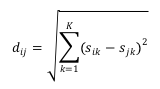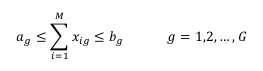# Maximally Diverse Grouping Problem

Gallego, Laguna, Martí and Duarte (2011)
Optsicom project, University of Valencia (Spain)

# Problem Description

The maximally diverse grouping problem (MDGP) consists of grouping a set of M elements into G mutually disjoint groups in such a way that the diversity among the elements in each group is maximized. The diversity among the elements in a group is calculated as the sum of the individual distance between each pair of elements, where the notion of distance depends on the specific application context. The objective of the problem is to maximize the overall diversity, i.e., the sum of the diversity of all groups. Feo and Khellaf (1990) proved that the MDGP is NP-hard.

In order to formulate the MDGP in mathematical terms, we assume that each element can be represented by a set of attributes. Let sik be the state or value of the kth attribute element, where k= 1,...,K and i=1,...,M. Then, the distance dij between element i and j may be simply defined by the Eucliden calculation:We have identified two variants of the MDGP. The first one (MDGP1) is the better known and forces all groups to have the same number of elements, with S=M/G. The second variant (MDGP2) allows the size Sg of each group g to be in the interval [ag,bg], where ag <= bg for g = 1,...,G. Clearly, MDGP1 is a special case of the MDGP2 for which ag = bg for all g.

Both variants can be formulated as quadratic integer programs with binary variables xig that take the value of 1 if element i is in group g and 0 otherwise. A quadratic integer programming formulation of the MDGP1 is:The objective function adds the distance of all pairs of elements that belong to the same group. The first set of constraints forces the assignment of each element to a group. The second set of constraints forces the size of all groups to be equal to S. In the more general case, MDGP2, the second set of constraints is replaced with:# State of the Art Methods

The most relevant approximated methods developed to solve this problem are:

• LCW: Multistart algorithm composed by a random constructive and an improvement method. The improvement method is developed as a modified version of LC method due to Lofti and Cerveny (1991). Weitz and Lakshminarayanan (1998)
• LSGA: Hybrid genetic algorithm that combines a genetic algorithm and a local search procedure. The genetic aspect of LSGA is based on the encoding scheme for grouping problems proposed by Falkenauer (1998). The local search within LSGA implements a best improvement strategy based on exchanging elements between groups. Fan et al. (2011)
• T‐LCW: Multistart algorithm composed by a greedy constructive (GC) and T-LCW, a tabu version of LCW improvement method. Gallego et al. (2011)
• SO: Multistart algorithm composed by a greedy constructive (GC) and strategic oscillation improvement based on T-LCW. Gallego et al. (2011)

A Java 6 implementation of all state of the art methods is available for download as binary. For usage instructions execute .jar file with the command:

```java -jar mdgp_jors_2011.jar
```

Please make sure that you have Java 6 SE installed in your system.

# Instances (MDGPLIB)

We have compiled a comprehensive set of 480 benchmark instances representative of the collections previously used for computational experiments in the MDGP. We call this benchmark MDPGLIB. Furthermore we have included new hard instances. A brief description of the origin and the characteristics of the set of instances follows:

• RanReal: This set consists of 160 M x M matrices in which the distance values dij are real numbers generated using a Uniform distribution U(0,100). The number of elements M, the number of groups G and the limits of each group ag and bg are shown in Table 1. There are 20 instances for each combination of parameters (i.e., each row in Table 1), 10 for instances with equal group size (EGS) and 10 for instances with different group size (DGS). For the 10 instances in EGS, the group size is equal for all instances and is calculated as ag = ag = M / G. For the 10 instances in DGS, the limits of each group (ag and bg) for each instance are generated randomly in the predefined interval. That is, the value of ag is generated in the interval [agmin,agmax] and the value of bg is generated in the interval [bgmin,bgmax]. This data set was introduced by Fan et al. (2011) with M ranging from 10 to 240. The larger instances with M=480 and M=960 had been generated for inclusion in MDGPLIB.
• M G EGS DGS
ag = ag agmin agmax bgmin bgmax
10 2 5 3 5 5 7
12 4 3 2 3 3 5
30 5 6 5 6 6 10
60 6 10 7 10 10 14
120 10 12 8 12 12 16
240 12 20 15 20 20 25
480 20 24 18 24 24 30
960 24 40 32 40 40 48
Table 1. Summary of parameters to generate problem instances
• RanInt: This set has the same structure and size as RanReal (shown in Table 1) but distances are generated with an integer Uniform distribution.
• Geo: This set follows the same structure and size as the previous two, however, values are calculated as Euclidean distances between pair of points with coordinates randomly generated in [0,10]. The number of coordinates for each point is generated randomly in the 2 to 21 range.

# Computational Experience

We have performed a computational comparison of the state of the art methods on 240 instances (5 EGS instances and 5 DGS instances for M=10 to 960). All methods were stopped using the same time limit, which varied according to problem size, as specified in Table 2.

M Time (seconds)
<= 60 1
120 3
240 20
480 120
960 600
Table 2. CPU time limits

In this experiment we compute for each instance and each method the relative deviation Dev (in percent) between the best solution value, Value, obtained with the method and the best known value of this instance, BestValue. For each method, we also report the number of instances #Best for which the method obtains the best value.

All algorithms were implemented in Java 6 and the experiment was performed in a Intel Core 2 Quad CPU Q 8300 with 6 GiB of RAM and Ubuntu 9.04 64 bits OS.

Method Dev #Best Score
LSGA 0.61% 82 339
LCW 1.01% 80 438
T-LCW 0.17% 141 108
SO 0.04% 192 55

# References

• Fan, Z. P., Y. Chen, J. Ma and S. Zeng (2011) "A hybrid genetic algorithmic approach to the maximally diverse grouping problem", Journal of the Operational Research Society, vol. 62, pp. 92‐99.
• Feo, T. and M. Khellaf (1990) "A class of bounded approximation algorithms for graph partitioning", Networks, vol. 20, pp. 181‐195.
• Lotfi V. and R. Cerveny (1991) "A final exam scheduling package", Journal of the Operational Research Society, vol. 42, pp. 205‐216.
• Weitz, R. R. and S. Lakshminarayanan (1998) "An empirical comparison of heuristic methods for creating maximally diverse groups", Journal of the Operational Research Society, vol. 49, no. 6, pp. 635‐646.
• Gallego, M., Laguna, M., Martí, R. and Duarte, A. (2011) "Tabu Search with Strategic Oscillation for the Maximally Diverse Grouping Problem", Journal of the Operational Research Society. Accepted. Download.